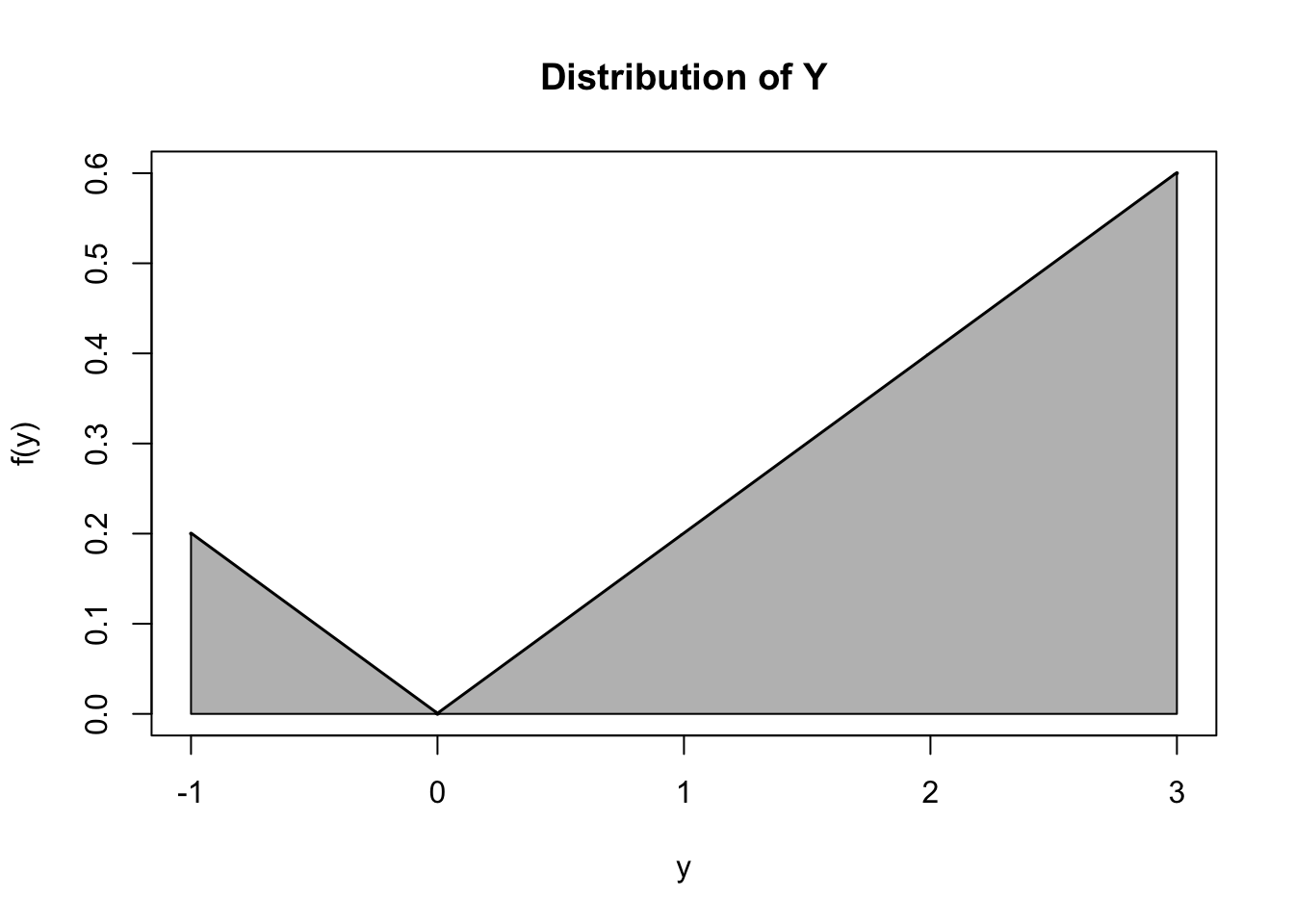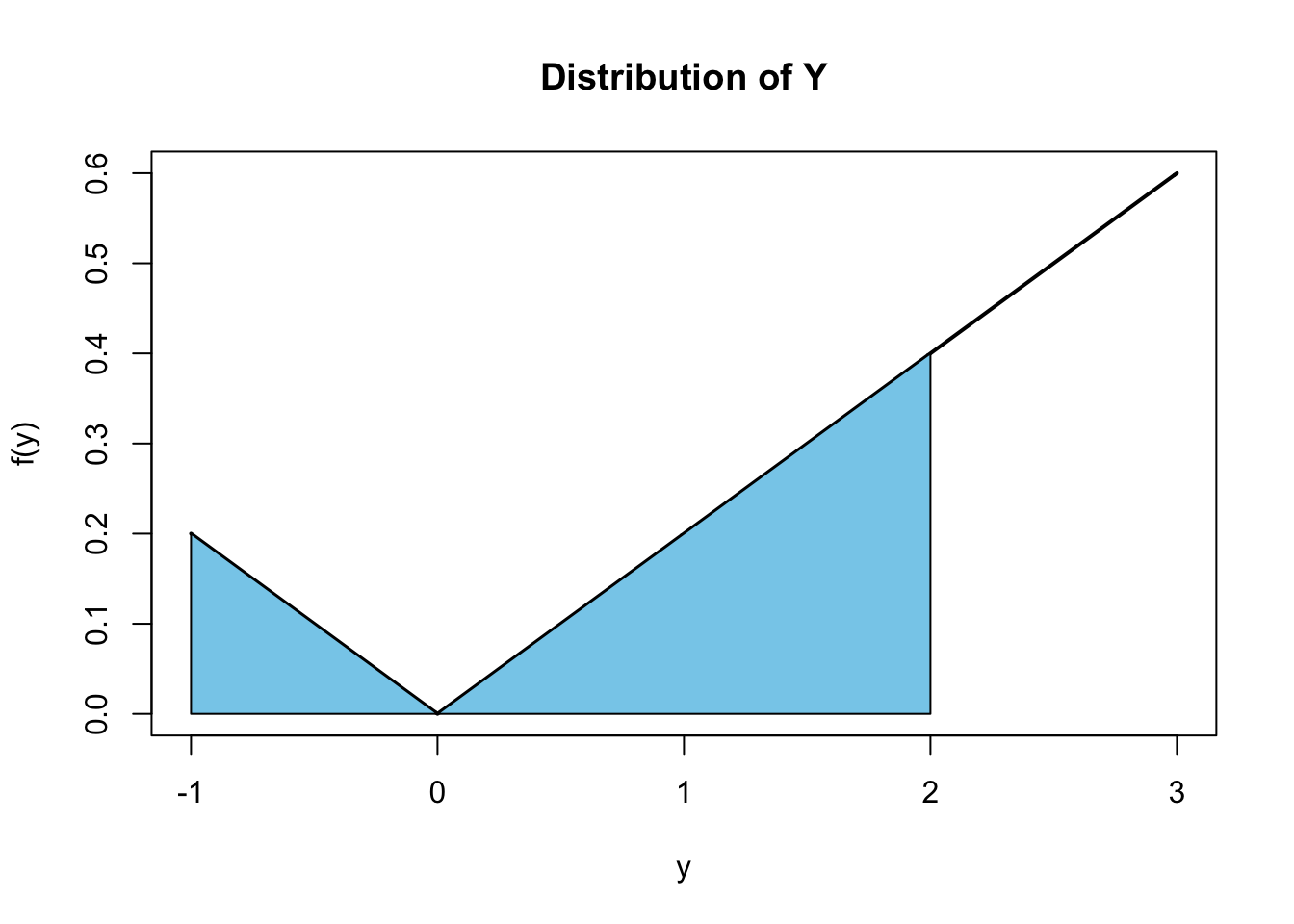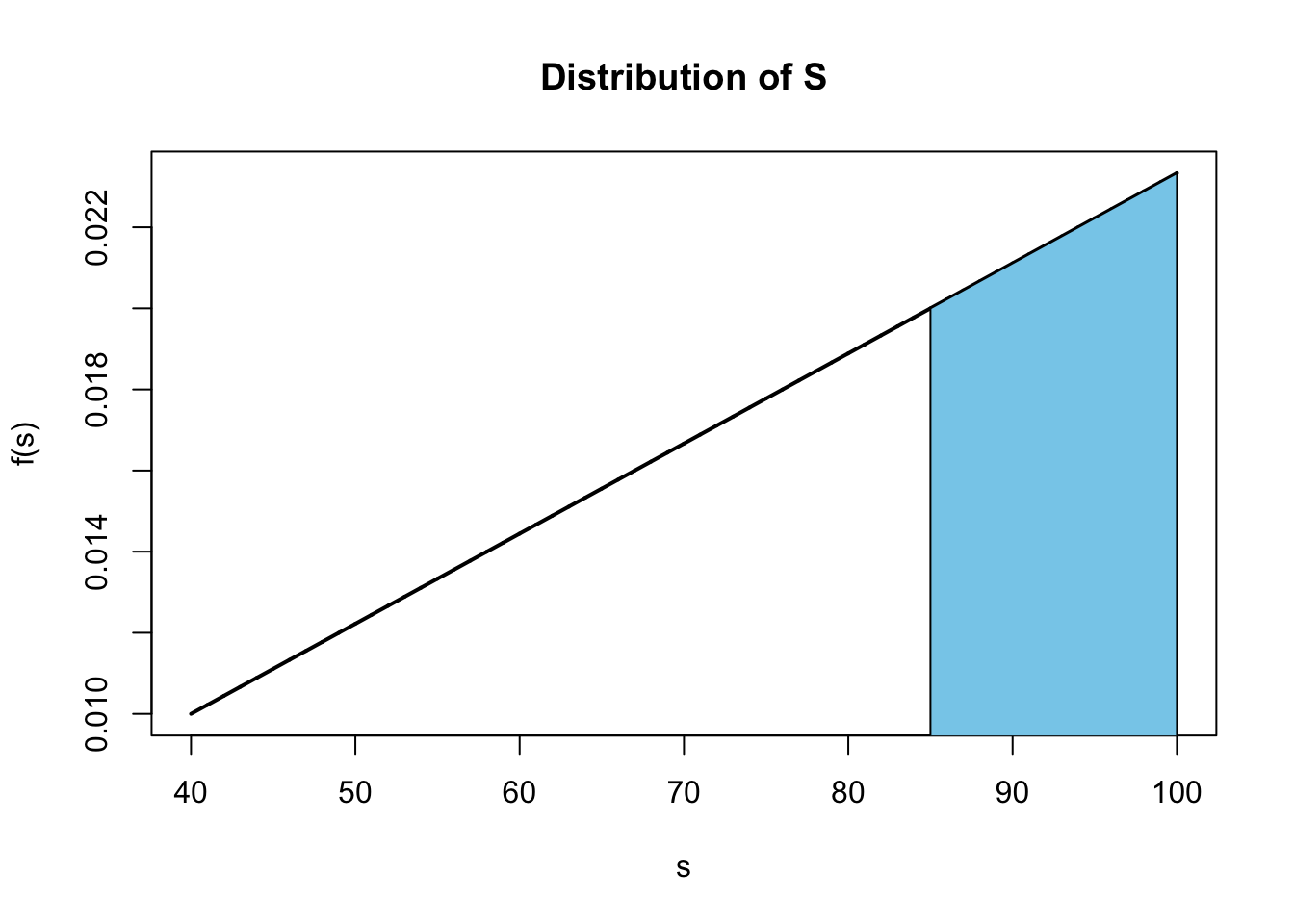## Exercise 1

Consider a random variable $$X$$ with the probability mass function

$f(x) = \frac{6}{3^x}, \quad x = 2, 3, 4, 5, \ldots$

(a) Find the moment-generating function of $$X$$, $$M_X(t)$$. Report the function, being sure to indicate the values of $$t$$ where the function exists.

Solution:

\begin{aligned} M_{X}(t) = \text{E}\left[e^{tX}\right] &= \sum_{x}^{} e^{tx} f(x) \\[0.5em] &= \sum_{x = 2}^{\infty} \frac{6e^{tx}}{3^x}\\[0.5em] &= 6 \sum_{x = 2}^{\infty} \left(\frac{e^t}{3}\right)^x\\[0.5em] &= 6 \cdot \left( \frac{\left(\frac{e^t}{3}\right)^2}{1 - \frac{e^t}{3}} \right)\\[0.5em] &= \boxed{\frac{2e^{2t}}{3 - e^t}} \ , \ \ \boxed{t < \log(3)} \end{aligned}

The restriction on $$t$$ is necessary since we require that $$\left|\frac{e^t}{3}\right|$$ be less than 1, otherwise the sum diverges, and the moment generating function does not exist.

(b) Calculate $$\text{E}[X]$$.

Solution:

$M'_{X}(t) = \frac{(3 - e^t)(4e^{2t}) - (2e^{2t})(-e^t)}{(3 - e^t)^2} = \frac{2e^{2t}(6 - e^t)}{(3 - e^t)^2}$

$\text{E}[X] = M'_{X}(0) = \frac{2(6 - 1)}{(3 - 1)^2} = \boxed{\frac{5}{2}}$

Alternatively, simply refer to Exercise 3 of Homework 3.

## Exercise 2

How much wood would a woodchuck chuck if a woodchuck could chuck wood? Let $$W$$ denote the amount of wood a woodchuck would chuck per day (in cubic meters) if a woodchuck could chuck wood. Suppose the moment-generating function of $$W$$ is

$M_W(t) = 0.1 \cdot e^{3t} + 0.3 \cdot e^{2t} + 0.5 \cdot e^{1t} + 0.1.$

(a) Calculate the average amount of wood a woodchuck would chuck per day, $$\text{E}[W]$$.

Solution:

Here, we use the moment generating function to generate the first moment, which is exactly the expected value.

$M'_{W}(t) = 0.3 \cdot e^{3t} + 0.6 \cdot e^{2t} + 0.5 \cdot e^{1t}$

$\text{E}[W] = M'_{W}(0) = \boxed{1.4}$

(b) Calculate $$\text{Var}[W]$$.

Solution:

Here, we use the moment generating function to generate the second moment.

$M''_{W}(t) = 0.9 \cdot e^{3t} + 1.2 \cdot e^{2t} + 0.5 \cdot e^{1t}$

$\text{E}[W^2] = M''_{W}(0) = 2.6$

We then calculate the variance by using the first and second moments that we had already calcualted.

$\text{Var}[W] = \text{E}[W^2] - \left(\text{E}[W]\right)^2 = 2.6 - 1.4^2 = \boxed{0.64}$

Alternatively, you could have realized that this moment generating function implies thet the probability mass function of $$W$$ is given by

$f(w) = \begin{cases} 0.1 & w = 0 \\ 0.5 & w = 1 \\ 0.3 & w = 2 \\ 0.1 & w = 3 \end{cases}$

then calculated the expected value and variance using the usual definitions. (Consider doing so for practice if you haven’t already.)

## Exercise 3

Consider a random variable $$Y$$ with the probability density function

$f(y) = \frac{|y|}{5}, \ -1 < y < 3.$

(a) Calculate $$\text{E}[Y]$$.Solution:

From the picture, it is clear that

$f(f) = \begin{cases} -0.2y & y < 0 \\ 0.2y & y \geq 0 \end{cases}$

Then we have

$\text{E}[Y] = \int_{-\infty}^{\infty} y \cdot f(y)dy = \int_{-1}^{0} y \cdot (-0.2y)dy + \int_{0}^{3} y \cdot (0.2y)dy = -\frac{1}{15} + \frac{9}{5} = \boxed{\frac{26}{15}}$

(b) Calculate $$\text{median}[Y]$$, the median of $$Y$$.Solution:

Here we need to find $$m$$ such that

$\int_{-\infty}^m f(y)dy = 0.5$

First, note that,

$\int_{-1}^{0} (-0.2y)dy = 0.1$

Thus, we know that $$m > 0$$.

Now, we need

$\int_{-1}^{0} (-0.2y)dy + \int_{0}^{m} (0.2y)dy = 0.5$

That is

$\int_{0}^{m} (0.2y)dy = 0.4$

Then finally, we have

$0.1 \cdot m ^ 2 = 0.4$

This implies that

$m = \boxed{2}$

since $$-2$$ is outside the possible values of the random variable.

## Exercise 4

Suppose that scores on the previous semester’s STAT 400 Exam II were not very good. Graphed, their distribution had a shape similar to the probability density function

$f(s) = \frac{1}{9000}(2s + 10), \ \ 40 \leq s \leq 100.$

Assume that scores on this exam, $$S$$, actually follow this distribution. (Note: This distribution does not necessarily reflect reality.)

(a) Suppose 10 students from the class are selected at random. What is the probability that (exactly) 4 of them received a score above 85?Solution:

Define $$B$$ to be the number of students how receive an 85 or above. Then $$B \sim \text{binom}(N = 10, p)$$ where

$p = \int_{85}^{100} \frac{1}{9000}(2s + 10)ds = \frac{s^2 + 10s}{9000}\Big|_{s = 85}^{s = 100} = \frac{11000}{9000} - \frac{8075}{9000} = 0.325$

$P(B = 4) = {10 \choose 4} (0.325)^4 (0.675)^6 \approx \boxed{0.2216}$

(b) What was the standard deviation of the scores, $$\text{SD}[S]$$?

Solution:

$\text{E}[S] = \int_{40}^{100} s \cdot \frac{2s + 10}{9000}\ ds = 74$

$\text{E}[S^2] = \int_{40}^{100} s^2 \cdot \frac{2s + 10}{9000}\ ds = 5760$

$\text{Var}[S] = \text{E}[S^2] - \left(\text{E}[S] \right) ^ 2 = 5760 - 74^2 = 284$

$\text{SD}[S] = \sqrt{\text{Var}[S]} = \sqrt{284} \approx \boxed{16.8523}$

(c) What was the class 40th percentile? That is, find $$a$$ such that $$P(S \leq a) = 0.40$$.